2018-12-19 17:33:12 RabitMountain 阅读数 128
• ###### 机器学习入门30天实战

购买课程后，可扫码进入学习群，获取唐宇迪老师答疑 系列课程包含Python机器学习库，机器学习经典算法原理推导，基于真实数据集案例实战3大模块。从入门开始进行机器学习原理推导，以通俗易懂为基础形象解读晦涩难懂的机器学习算法工作原理，案例实战中使用Python工具库从数据预处理开始一步步完成整个建模工作！具体内容涉及Python必备机器学习库、线性回归算法原理推导、Python实现逻辑回归与梯度下降、案例实战，信用卡欺诈检测、决策树与集成算法、支持向量机原理推导、SVM实例与贝叶斯算法、机器学习常规套路与Xgboost算法、神经网络。

7605 人正在学习 去看看 唐宇迪

## 机器学习实战（1）—— 机器学习基础### 1.2 关键术语

1 1000.1 125.0 棕色 红尾鵟
2 3000.7 200.0 灰色 鹭鹰
3 3300.0 220.3 灰色 鹭鹰
4 4100.0 136.0 黑色 普通潜鸟
5 3.0 11.0 绿色 瑰丽蜂鸟
6 570.0 75.0 黑色 象牙喙啄木鸟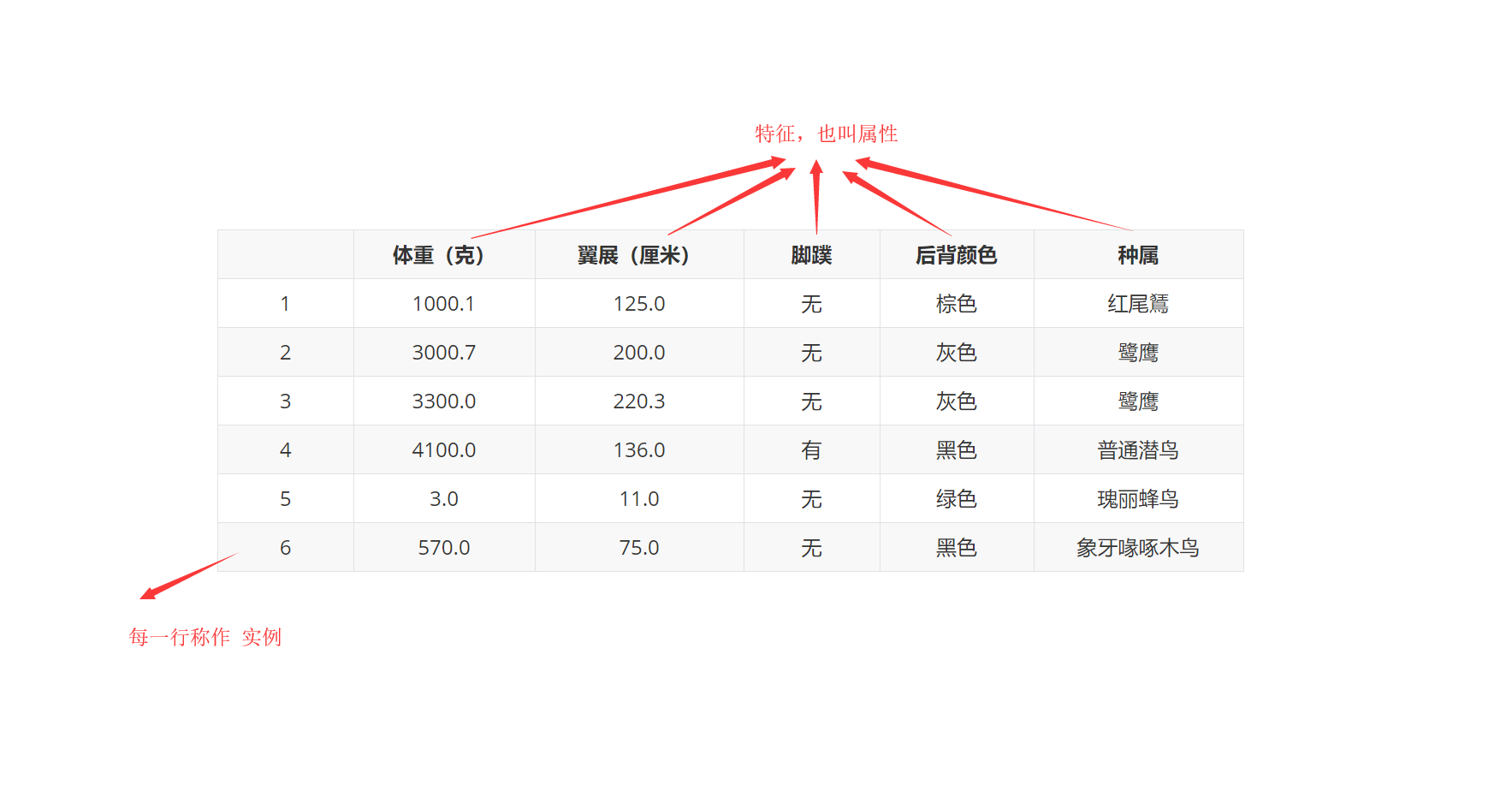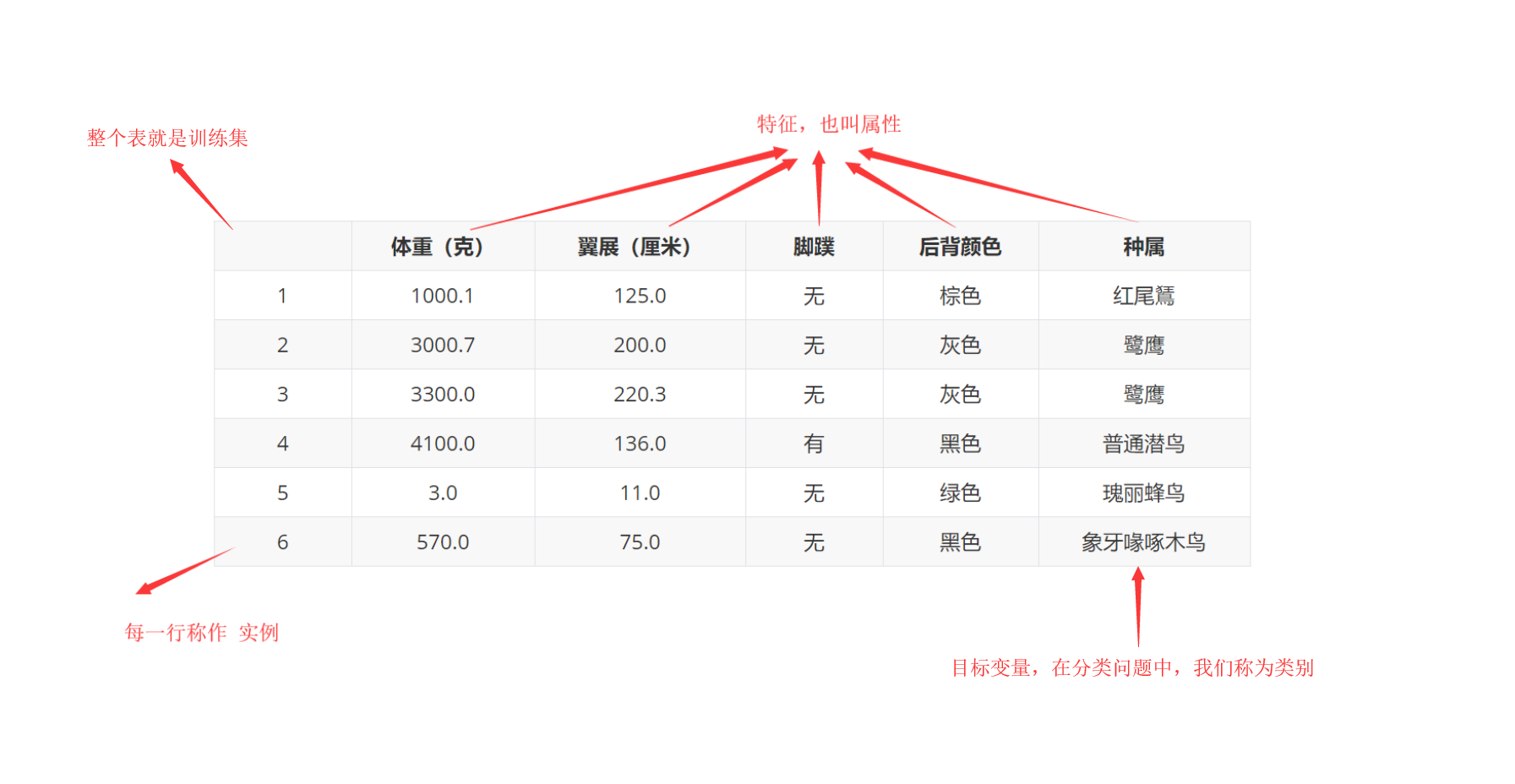1. 当机器学习程序开始运行时，使用训练样本集作为算法的输入，训练完成之后输入测试样本。
2. 输入测试样本时并不提供测试样本的目标变量，由程序决定样本属于哪个类别。比较测试样本预测的目标变量值与实际样本类别之间的差别，就可以得出算法的实际精确度。

1. 实例（样本）：表中每一行是关于一个事件或者对象的描述。
2. 特征（feature）：反映事件或对象在某方面的表现或性质的事项，也叫做属性（attribute），例如体重、是否有脚蹼等。
3. 属性值（attribute value）:属性上的取值。
4. 数据集（data set）：样本的集合。
5. 训练集（training set）：用作训练的数据集。
6. 测试集（test set）：用作测试的数据集。

### 1.3 机器学习的主要任务

1. 将数据集合分成由类似的对象组成的多个类的过程被称为聚类
2. 将寻找描述数据统计值 的过程称之为密度估计

k-近邻算法 线性回归

K-均值 最大期望算法
DBSCAN Parzen窗设计

### 1.4 如何选择合适的算法

1. 我们使用机器学习算法的目的，想要算法完成何种任务。比如，是预测明天下雨的概率还是对投票者按照兴趣分组呢？
2. 需要分析和收集的数据是什么

**首先考虑使用机器学习算法的目的。**如果想要预测目标变量的值，则可以选择监督学习算法， 否则可以选择无监督学习算法。确定选择监督学习算法之后，需要进一步确定目标变量类型，如果目标变量是离散型，如是/否、1/2/3、A/B/C或者红/黄/黑等，则可以选择分类器算法；如果目标变量是连续型的数值，如0.0～100.00、-999～999或者+∞～-∞等，则需要选择回归算法。 如果不想预测目标变量的值，则可以选择无监督学习算法。进一步分析是否需要将数据划分为离散的组。如果这是唯一的需求，则使用聚类算法；如果还需要估计数据与每个分组的相似程度，则需要使用密度估计算法。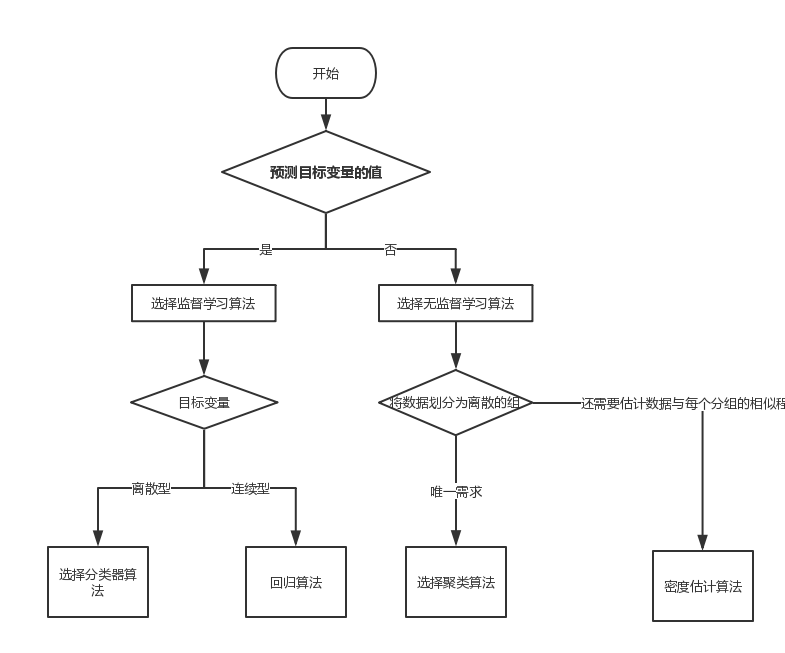1. 特征值是离散型变量还是连续型变量
2. 特征值中是否存在缺失的值
3. 何种原因造成缺失值
4. 数据中是否存在异常值
5. 某个特征发生的频率如何（是否罕见得如同海底捞针）等等。

### 1.5 开发机器学习应用程序的步骤

2. 准备输入数据。得到数据之后，还必须确保数据格式符合要求。在这本书中采用的格式是Python语言的List。使用这种标准数据格式可以融合算法和数据源，方便匹配操作。此外还需要为机器学习算法准备特定的数据格式，如某些算法要求特征值使用特定的格式， 一些算法要求目标变量和特征值是字符串类型，而另一些算法则可能要求是整数类型。
3. 分析输入数据。此步骤主要是人工分析以前得到的数据。为了确保前两步有效，简单的方法是用文本编辑器打开数据文件，查看得到的数据是否为空值。此外，还可以进一步浏览数据，分析是否可以识别出模式；数据中是否存在明显的异常值，如某些数据点与数据集中的其他 值存在明显的差异。通过一维、二维或三维图形展示数据也是不错的方法，然而大多数时候我们 得到数据的特征值都不会低于三个，无法一次图形化展示所有特征。本书的后续章节将会介绍提 炼数据的方法，使得多维数据可以压缩到二维或三维，方便我们图形化展示数据。 这一步的主要作用是确保数据集中没有垃圾数据。如果是在产品化系统中使用机器学习算法 并且算法可以处理系统产生的数据格式，或者我们信任数据来源，可以直接跳过第3步。此步骤需要人工干预，如果在自动化系统中还需要人工干预，显然就降低了系统的价值。
4. 训练算法。机器学习算法从这一步才真正开始学习。根据算法的不同，第4步和第5步是机器学习算法的核心。我们将前两步得到的格式化数据输入到算法，从中抽取知识或信息。这里 得到的知识需要存储为计算机可以处理的格式，方便后续步骤使用。 如果使用无监督学习算法，由于不存在目标变量值，故而也不需要训练算法，所有与算法相关的内容都集中在第5步。
5. 测试算法。这一步将实际使用第4步机器学习得到的知识信息。为了评估算法，必须测试算法工作的效果。对于监督学习，必须已知用于评估算法的目标变量值；对于无监督学习，也必须用 其他的评测手段来检验算法的成功率。无论哪种情形，如果不满意算法的输出结果，则可以回到第 4步，改正并加以测试。问题常常会跟数据的收集和准备有关，这时你就必须跳回第1步重新开始。
6. 使用算法。将机器学习算法转换为应用程序，执行实际任务，以检验上述步骤是否可以 在实际环境中正常工作。此时如果碰到新的数据问题，同样需要重复执行上述的步骤。2019-03-27 14:25:01 gzyiCG 阅读数 160
• ###### 机器学习入门30天实战

购买课程后，可扫码进入学习群，获取唐宇迪老师答疑 系列课程包含Python机器学习库，机器学习经典算法原理推导，基于真实数据集案例实战3大模块。从入门开始进行机器学习原理推导，以通俗易懂为基础形象解读晦涩难懂的机器学习算法工作原理，案例实战中使用Python工具库从数据预处理开始一步步完成整个建模工作！具体内容涉及Python必备机器学习库、线性回归算法原理推导、Python实现逻辑回归与梯度下降、案例实战，信用卡欺诈检测、决策树与集成算法、支持向量机原理推导、SVM实例与贝叶斯算法、机器学习常规套路与Xgboost算法、神经网络。

7605 人正在学习 去看看 唐宇迪

提前准备
Python
Jupyter笔记本



Jupyter笔记本也是常用的工具，不用下载，在Web上就能直接用，可以在线coding，许多重要的应用和教程也是在Jupyter上的，一定要学会。

用Scikit-Learn做机器学习



TensorFlow与神经网络

TensorFlow启动并运行
ANN - 人工神经网络
CNN - 卷积神经网络
RNN - 循环神经网络



一些实用资料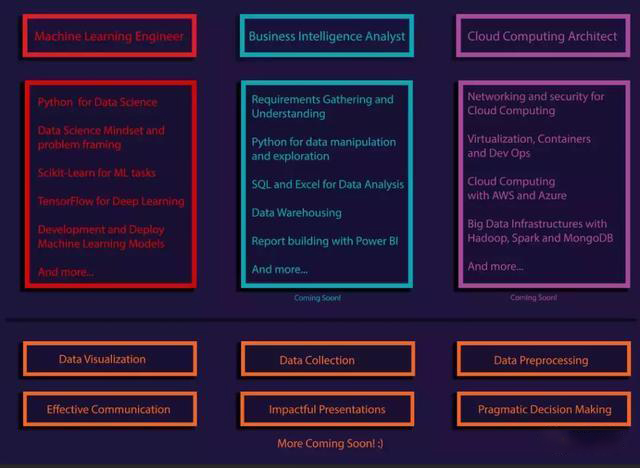欢迎大家加入小编创建的Python行业交流群，有大牛答疑，有资源共享，



2018-04-16 16:30:56 yatou2016 阅读数 2650
• ###### 机器学习入门30天实战

购买课程后，可扫码进入学习群，获取唐宇迪老师答疑 系列课程包含Python机器学习库，机器学习经典算法原理推导，基于真实数据集案例实战3大模块。从入门开始进行机器学习原理推导，以通俗易懂为基础形象解读晦涩难懂的机器学习算法工作原理，案例实战中使用Python工具库从数据预处理开始一步步完成整个建模工作！具体内容涉及Python必备机器学习库、线性回归算法原理推导、Python实现逻辑回归与梯度下降、案例实战，信用卡欺诈检测、决策树与集成算法、支持向量机原理推导、SVM实例与贝叶斯算法、机器学习常规套路与Xgboost算法、神经网络。

7605 人正在学习 去看看 唐宇迪1.python基础篇教程（22课）2.python入门与进阶1.编程入门

（1）matlab与机器学习入门 进阶和提高 （共13课时，内附练习、matlab代码和算法包，还有ppt课件）（2）深度学习 tensorflow教程（是莫烦的教学视频，最大特点是简单易懂）2.基础精讲

（1）原理精讲班 （共12课时）（2）基础一（个人觉得挺不错的 ，讲的很详细）（3）基础二（紧接着基础一）3.深度学习

（1）深度神经网络深入研究 （共20课时，内附ppt课件）（2）深度神经网络深入与强化 （共10课时，内附ppt课件）2018-09-14 11:26:41 wuzhiwuweisun 阅读数 473
• ###### 机器学习入门30天实战

购买课程后，可扫码进入学习群，获取唐宇迪老师答疑 系列课程包含Python机器学习库，机器学习经典算法原理推导，基于真实数据集案例实战3大模块。从入门开始进行机器学习原理推导，以通俗易懂为基础形象解读晦涩难懂的机器学习算法工作原理，案例实战中使用Python工具库从数据预处理开始一步步完成整个建模工作！具体内容涉及Python必备机器学习库、线性回归算法原理推导、Python实现逻辑回归与梯度下降、案例实战，信用卡欺诈检测、决策树与集成算法、支持向量机原理推导、SVM实例与贝叶斯算法、机器学习常规套路与Xgboost算法、神经网络。

7605 人正在学习 去看看 唐宇迪

QT1:

But.......................经过两天的折腾，博主的心态崩了，时间很宝贵，不能在这上边浪费时间了，老板还有任务呢。于是上网去查怎么 安装没有GPU加速的CPU版本Pytorch,成功安装。下一步就是安装scikit learn库了，scikit learn库需要下面几个依赖库的支持：

• Python (>= 2.6 or >= 3.3)
• NumPy (>= 1.6.1)
• SciPy (>= 0.9)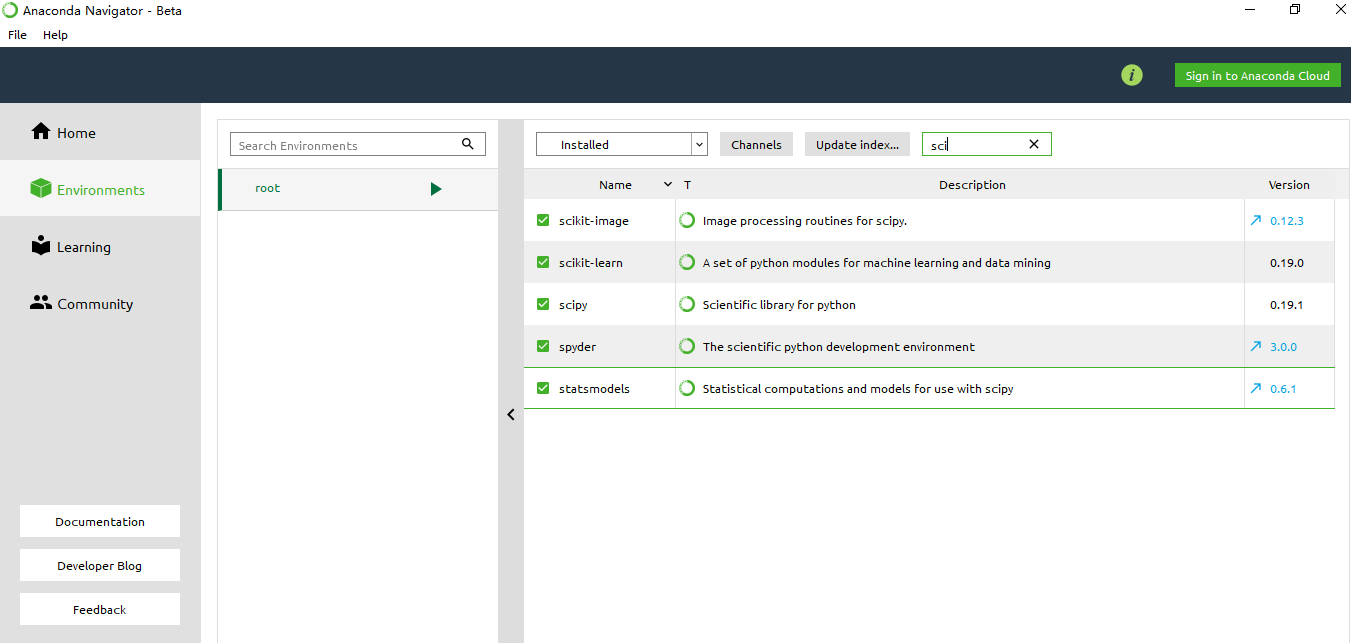QT2:

scikit-learn目前只支持cpu，不支持gpu。

1.scikit-learn不包括深度神经网络模型，这让scikit-learn有条件不基于GPU加速。

2.scikit-learn的设计目标是跨平台、易于安装，这让scikit-learn有意识地不基于GPU加速。

sklearn到底有没有深度神经网络模型，前边已经说没有了，然后这篇知乎给出了更具体的答案。

https://blog.csdn.net/gamer_gyt/article/details/51255448

### 附：安装sklearn链接

2.简书上比较详细的教程：https://www.jianshu.com/p/4e772ae08a4c

3.简书上比较详细的教程：https://www.jianshu.com/p/21b758541825

5.前面提到的依赖库，包括windows下最常用的其他依赖库的安装包都有！！！网址：https://www.lfd.uci.edu/~gohlke/pythonlibs/

6.最直接最方便的是Anaconda，都给你装好了。

1  >>> import sklearn
2  >>> sklearn.__version__
3  '0.17.1'

2019-09-09 20:06:19 qq_38418700 阅读数 42
• ###### 机器学习入门30天实战

购买课程后，可扫码进入学习群，获取唐宇迪老师答疑 系列课程包含Python机器学习库，机器学习经典算法原理推导，基于真实数据集案例实战3大模块。从入门开始进行机器学习原理推导，以通俗易懂为基础形象解读晦涩难懂的机器学习算法工作原理，案例实战中使用Python工具库从数据预处理开始一步步完成整个建模工作！具体内容涉及Python必备机器学习库、线性回归算法原理推导、Python实现逻辑回归与梯度下降、案例实战，信用卡欺诈检测、决策树与集成算法、支持向量机原理推导、SVM实例与贝叶斯算法、机器学习常规套路与Xgboost算法、神经网络。

7605 人正在学习 去看看 唐宇迪

# 前端实现机器学习

## 再说怎么做

### python代码

// python代码
import numpy as np

def sigmoid(z):
sigmoid = 1.0 / (1.0 + np.exp(-z))
return sigmoid

# 梯度方向
gradient = np.dot(X.T, (h - y)) / y.shape

# 逻辑回归过程
def Logistic_Regression(x, y, lr=0.05, count=200):
intercept = np.ones((x.shape, 1)) # 初始化截距为 1
x = np.concatenate((intercept, x), axis=1)
w = np.zeros(x.shape) # 初始化参数为 0
for i in range(count): # 梯度下降迭代
z = np.dot(x, w) # 线性函数
h = sigmoid(z)
g = gradient(x, h, y) # 计算梯度
w -= lr * g # 通过学习率 lr 计算步长并执行梯度下降

return w # 返回迭代后的梯度和参数
//这是我手动选择色块得到的假数据，为什么是三维呢？因为我只存了两个色块三原色的差值
x = np.array([
[-255, -255, -255],
[-12, 43, -89],
[-42, -25, 102],
[7, 202, 81],
[142, -67, -120],
[100, 52, -6],
[-19, -41, -24],
[-108, 179, 78],
[-192, 166, 27],
[80, -30, -47],
[53, 38, 140]
])
y = np.array([1, 1, 0, 0, 1, 0, 1, 0, 0, 1, 0])
w = Logistic_Regression(x, y)


### javascript代码

// js代码
// 这个是假数据，注释掉了，因为把它整体封装成了一个方法
// const x = [
//     [-255, -255, -255],
//     [-12, 43, -89],
//     [-42, -25, 102],
//     [7, 202, 81],
//     [142, -67, -120],
//     [100, 52, -6],
//     [-19, -41, -24],
//     [-108, 179, 78],
//     [-192, 166, 27],
//     [80, -30, -47],
//     [53, 38, 140]
// ]
// const y = [1, 1, 0, 0, 1, 0, 1, 0, 0, 1, 0]
// const z = [-30, 100, -66]
// 入参x是之前色块的数据矩阵（条件），y是选择矩阵（结果），z是最后选择时色块的色差（最新条件）
export const MySigmod = function(x, y, z) {
//sigmod函数
const sigmod = function(z) {
let c = []
for(let i = 0; i < z.length; i++) {
c.push(1/(1+Math.exp(-z[i])))
}
return c
}
//梯度方向
const gradient = function(x, h, y) {
let g = []
for(let j = 0; j < x.length; j++) {
let c = 0
for(let i = 0; i < y.length; i++) {
c = c + x[i][j] * (h[i] - y[i])
}
c = c / y.length
g.push(c)
}
return g
}
//逻辑回归过程
function Logistic_Regression(x, y, lr=0.05, count=500) {
let w = []
x.map(item => {
item.push(1)
})
for(let i = 0; i < x.length; i++) {
w.push(0)
}
for(let m = 0; m < count; m++) {
let z = []
for(let i = 0; i < x.length; i++) {
let item = 0
for(let j = 0; j < w.length; j++) {
item = item + x[i][j] * w[j]
}
z.push(item)
}
let h = sigmod(z)
let g = gradient(x, h, y)
for(let i = 0; i < w.length; i++) {
w[i] = w[i] - lr * g[i]
}
// l = loss(h, y)
}
return w
}

let w = Logistic_Regression(x,y)
//使用求出的权重系数尽心选择
let p = w
for(let i = 0; i < z.length; i++) {
p = p + z[i] * w[i]
}
p = 1/(1+Math.exp(p))
return p
}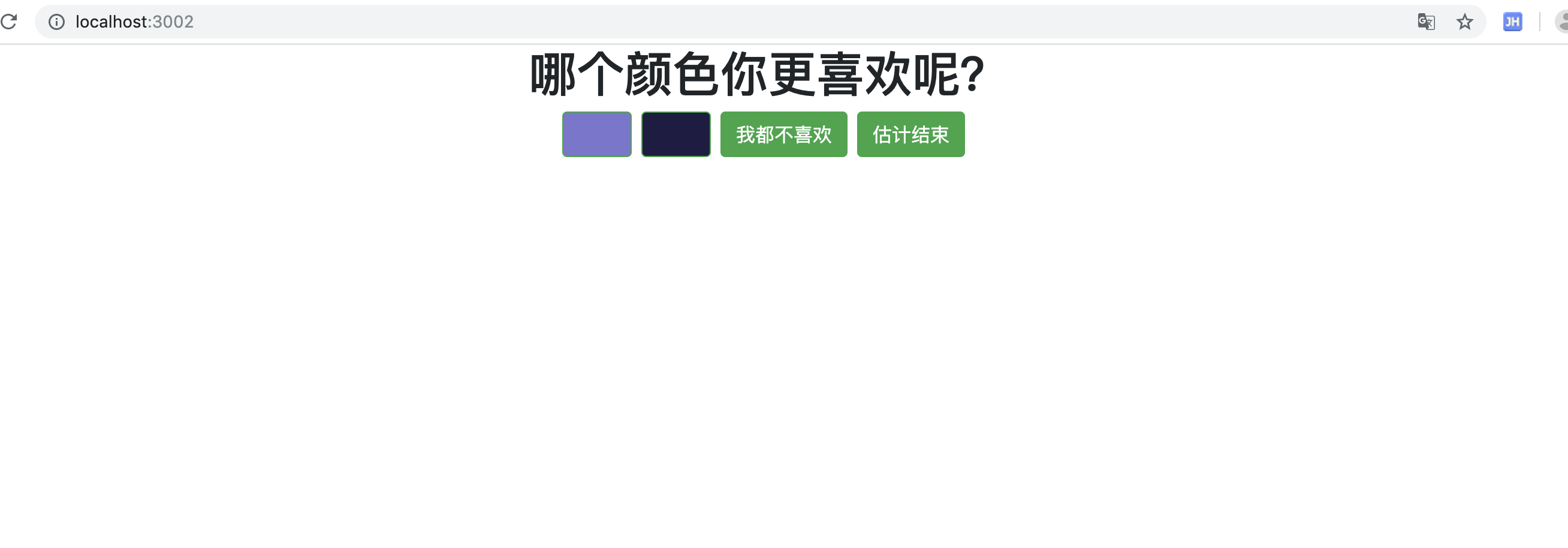## 再说为什么

$g(z) = \frac{1}{(1+e^{-z})}\quad$

$z = w_0x_0+w_1x_1+w_2x_2+....+w_nx_n$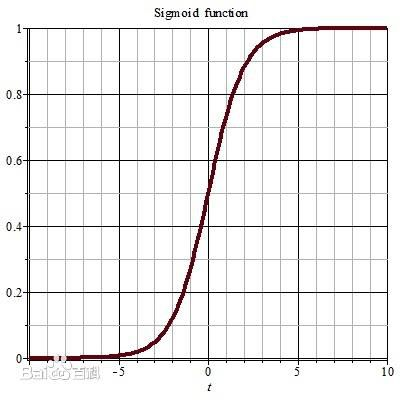$\quad \prod_{i=1}^m p(y_i|x_i,\theta)$

$L(\theta)=\quad \prod_{i=1}^m p(y_i|x_i,\theta)=\quad \prod_{i=1}^mg(z_i)^{y_i} (1-g(z_i))^{1-y_i}$

$l (\theta)= \sum_{i=1}^m y_i\log g(z_i) +(1-y_i)\log (1-g(z_i))$

$l (\theta_j)= -\frac{1}{m}\quad \sum_{i=1}^m (z_i-y_i)*x_{ij}$